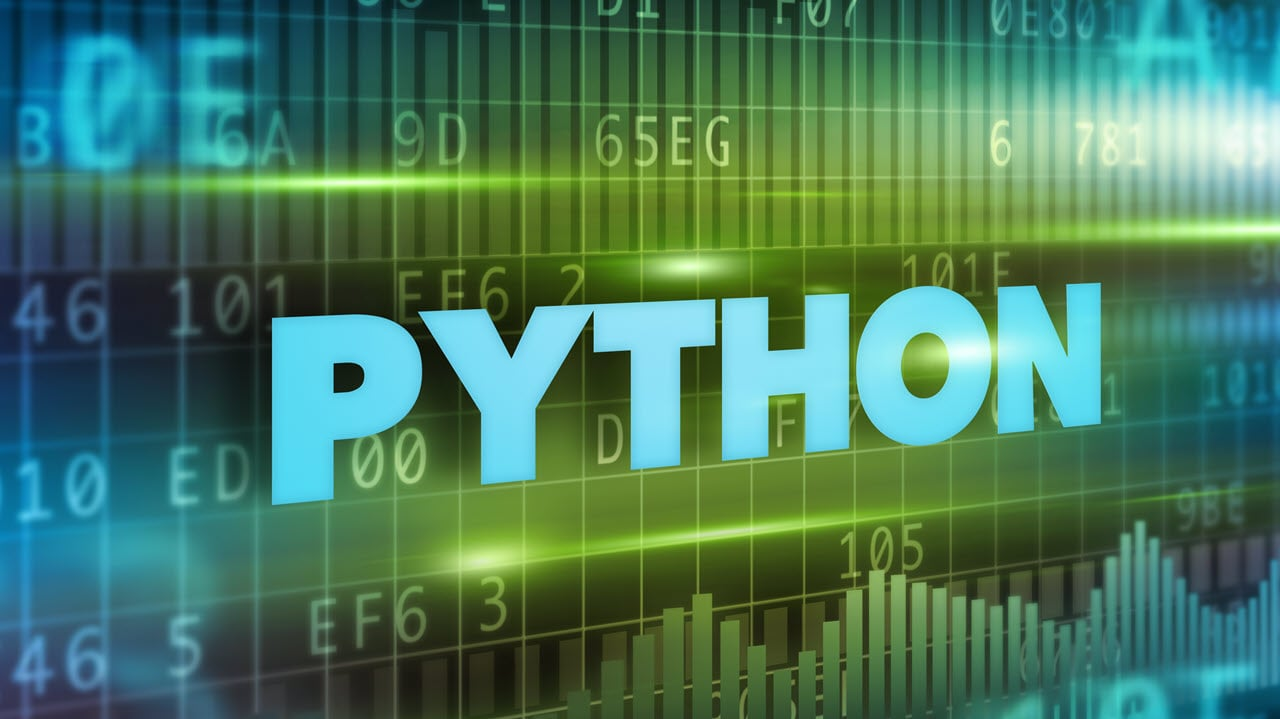# How to remove first element from array in Python?Python is one of the easiest programming languages for complete beginners to learn. However, while it might be easy to get to grips with, it’s still quite powerful and allows developers to make anything from simple web scraping scripts to powerful AI or ML programs.

A lot of this ‘power’ comes from the different types of data structures that Python supports. In this article, we’re talking about how to remove the first element from an array in Python.

# Arrays in Python

Since Python doesn’t have built-in support for Arrays, it uses lists instead. While both of them might sound familiar, it’s far easier to work with lists in Python considering the built-in support and functionality.

# Removing the first element in a list

There are five methods you can use to remove the first or any other element from a list in Python:

• Using pop()
• Using del list[]
• Python Slicing
• Using deque() and popleft()
• Using remove()

## Using pop()

The pop() method removes and prints the specified element from a list. The general syntax is as follows.

``list_name.pop(x) where x is the element position. ``

To remove the first element of the list, use position 0 as shown in the snippet below.

``````# Initialising list
test_list = [1, 2, 3, 4, 5]

# Using pop(0) to remove the first element
test_list.pop(0)

#Printing updated list
print("Updated list: " + str(test_list))``````

## Using del[]

A method similar to pop, del also removes the specified element from a list. In order to delete the first element, use position zero. The general syntax is as follows.

``list_name.del[x] where x is the element position.``

To remove the first element of the list, use position 0 as shown in the snippet below.

``````# Initialising list
test_list = [1, 2, 3, 4, 5]

# Using del list to remove the first element
del test_list

# Printing updated list
print("Updated list: " + str(test_list))
``````

## Using Python Slicing

In slicing, the specified element is deleted and the list is transferred to a new variable. Here’s how you would use slicing to remove the first element in a list.

``````# Initialising list
test_list = [1, 2, 3, 4, 5]

# Using slicing to remove the first element
res = test_list[1:]

# Printing updated list
print("Updated list: " + str(res))
``````

## Using deque() and popleft()

Converting the list into deque and then using popleft will also remove the first element of a list. Here’s how you would use the two methods.

``````# Importing deque
from collections import deque

# Initialising list
test_list = [1, 2, 3, 4, 5]

# Using deque() + popleft() to
# remove the first element
res = deque(test_list)
res.popleft()

# Printing updated list
print("Updated list: " + str(list(res)))
``````

## Using remove()

Similar to the pop and del functions, remove also removes the specified variable from a list. The general syntax is as follows.

``list_name.remove(list_name[x]) where x is the element position.``

To remove the first element of the list, use position 0 as shown in the snippet below.

``````# Initialising list
test_list = [1, 2, 3, 4, 5]

# Remove first element from list
test_list.remove(test_list)

# Printing updated list
print("Updated list: " + str(test_list))``````